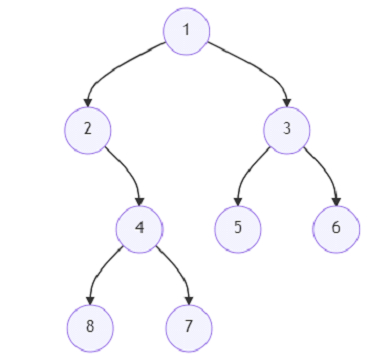# Program to find number of good leaf nodes pairs using Python

Suppose we have a binary tree. and another value distance d. A pair of two different leaf nodes are said to be good, when the shortest path between these two nodes is smaller or same as distance d.

So, if the input is likeAnd distance d = 4, then the output will be 2 because the pairs are (8,7) and (5,6) as their path length distance is 2, but (7,5) or (8,6) or other pairs are not good as their path length is 5 which is larger than d = 4

To solve this, we will follow these steps −

• sol := 0

• Define a function util() . This will take root

• if root is null, then

• return a new list

• if root is leaf, then

• return an array with one pair [0, 0]

• otherwise,

• cur := a new list

• l := util(left of root)

• r := util(right of root)

• for each n in l, do

• n := n + 1

• for each n in r, do

• n := n + 1

• for each n in r, do

• for each n1 in l, do

• if n + n1 <= d, then

• sol := sol + 1

• return l+r

• From the main method do the following −

• util(root)

• return sol

Let us see the following implementation to get better understanding −

## Example

Live Demo

class TreeNode:
def __init__(self, val=0, left=None, right=None):
self.val = val
self.left = left
self.right = right
class Solution:
def __init__(self):
self.sol = 0
def solve(self, root, d):
def util(root):
if not root:
return []
if not root.left and not root.right:
return [[0, 0]]
else:
cur = []
l = util(root.left)
r = util(root.right)
for n in l:
n += 1
for n in r:
n += 1
for n in r:
for n1 in l:
if n + n1 <= d:
self.sol += 1
return l+r
util(root)
return self.sol
root = TreeNode(1)
root.left = TreeNode(2)
root.right = TreeNode(3)
root.left.right = TreeNode(4)
root.left.right.left = TreeNode(8)
root.left.right.right = TreeNode(7)
root.right.left = TreeNode(5)
root.right.right = TreeNode(6)
d = 4
ob = Solution()
print(ob.solve(root, d))

## Input

root = TreeNode(1)
root.left = TreeNode(2)
root.right = TreeNode(3)
root.left.right = TreeNode(4)
root.left.right.left = TreeNode(8)
root.left.right.right = TreeNode(7)
root.right.left = TreeNode(5)
root.right.right = TreeNode(6)
d = 4

## Output

2